# College Chemistry : Molecules and Compounds

## Example Questions

### Example Question #9 : Molecular Geometries And Vsepr Theory

What is the molecular geometry of the molecule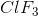?

Trigonal bipyramidal

T-shaped

Square pyramidal

Trigonal planar

Trigonal pyramidal

T-shaped

Explanation:is t-shaped. By drawing the Lewis diagram for, we see that there are 5 electron groups and 2 lone pairs on the central atom,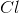. Electron group geometry states that molecules with 5 electron groups have a trigonal bipyramidal geometry. The molecular geometries of trigonal bipyramidal molecules can be trigonal bipyramidal (no lone pairs), seesaw (1 lone pair), t-shaped (2 lone pairs). and linear (3 lone pairs).consists of 5 electron groups and 2 lone pairs and therefore fits the molecular geometry of a t-shaped molecule.

### Example Question #10 : Molecular Geometries And Vsepr Theory

Which of the following is true of both molecular orbitals and atomic orbitals?

Molecular orbitals are to valence bond theory as atomic orbitals are to atomic orbital theory.

The number of atomic orbitals are twice the number of molecular orbitals for a given molecule.

The number of molecular orbitals are half the number of atomic orbitals for a given molecule.

No comparison between molecular orbitals and atomic orbitals can be made as they are consistent with different theories of bonding.

The number of molecular orbitals is equal to the number of atomic orbitals for a given molecule.

The number of molecular orbitals is equal to the number of atomic orbitals for a given molecule.

Explanation:

The number of molecular orbitals is equal to the number of atomic orbitals for a given molecule. The number of orbitals is consistent. Molecular orbitals shows destructive and constructive interference between sigma and pi bonds. In molecular orbital diagrams, these results appear as either bonding or anti bonding energies. Atomic orbitals combine the valence shells of overlapping orbitals to form more stable electron configurations.

### Example Question #11 : Molecular Geometries And Vsepr Theory

What is the hybridization of a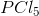molecule?

sp3d

sp3d2

sp4

sp2

sp

sp3d

Explanation:

The correct hybridization of ais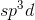(trigonal bipyramidal). By drawing a Lewis diagram for amolecule, we find 5 electron groups. Therefore,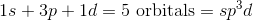### Example Question #1 : Nomenclature And Functional Groups

Name the compound: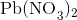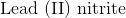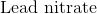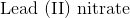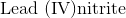Explanation:

First, recognize that this is an ionic compound.

Identify the cation and the anion. The cation is lead, and the anion is nitrate.

Since lead can form more than one type of ion, figure out the charge associated with the given cation.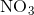has a charge of. Since there arenitrate ions, the charge for lead must be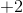. Remember to put the charge of the cation in parentheses when naming the compound.

The name of this compound is then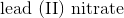.

### Example Question #2 : Nomenclature And Functional Groups

What is the correct name for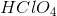?

Chlorous acid

Hydrochloric acid

Perchloric acid

None of these

Chloric acid

Perchloric acid

Explanation:consists of a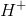ion bonded to a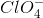ion, which is a perchlorate ion. Thus, the name of the ion's corresponding acid is perchloric acid.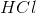is the molecular formula for hydrochloric acid,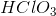is the formula for chloric acid, while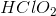is the formula for chlorous acid.

### Example Question #3 : Nomenclature And Functional Groups

Consider the following the trend of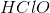,,, and. Identify the name of the ternary acid,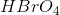.

Perbromic acid

Hypobromic acid

Hydorbromic acid

Bromous acid

Hypobromous acid

Perbromic acid

Explanation:

Because there are four oxygen atoms like in perchloric acid, the correct name is perbromic acid. Hypobromic acid is not a valid name for an acid since the "hypo-" prefix refers to one or two oxygen atoms but the "-ic" suffix refers to either three or four oxygen atoms. Hypobromous and bromous acid refer to one and two oxygen atoms, respectively. Hydrobromic acid has no oxygen atoms.

### Example Question #4 : Nomenclature And Functional Groups

What is the correct nomenclature for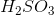?

Hydrogen sulfate

Hydrogen sulfide

Sulfurous acid

Sulfuric acid

Hydrogen sulfite

Sulfurous acid

Explanation:

The correct nomenclature foris sulfurous acid. Oxyanions ending in -ate form oxyacids ending in -ic. For example, sulfate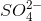becomes sulfuric acid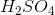. Oxyanions ending in -ite form oxyacids ending in -ous. In this problem, sulfite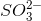becomes sulfurous acid.

### Example Question #5 : Nomenclature And Functional Groups

What is the correct molecular formula for cobalt (II) sulfite?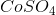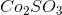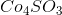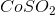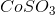Explanation:

The correct molecular formula for cobalt (II) sulfite is. The charge on cobalt (II) is. When combined with sulfite, the positive and negative charges cancel out to create a neutral compound.

### Example Question #31 : Compounds And Molecules

What is the oxidation state of manganese (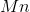) in the polyatomic permanganate anion (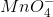)?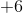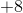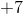Explanation:

When assigning oxidation states to elements of a given compound, non-transition metal elements are assigned specific oxidation states corresponding to their group number and valence relative to a complete octet.

Group 1 elements have 1 valence electron and an oxidation state of +1.

Group 2 elements have 2 valence electrons and an oxidation state of +2.

Group 8 elements (the noble gases) have a complete octet, thus their assigned oxidation state is 0.

Group 7 elements (halogens) have 7 valence electrons and an oxidation state of -1.

Group 6 elements such as oxygen have 6 valence electrons and an oxidation state of -2.

Permanganate has 4 oxygen atoms and an overall charge of -1. The oxidation state of theatom may be found by first calculating the combined oxidation state of the oxygen atoms: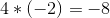and finding the difference between their combined oxidation state and the overall charge of the ion (-1):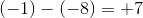### Example Question #32 : Compounds And Molecules

According to VSEPR theory, for the ammonium ion (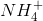), the electron-pair geometry is __________ and the molecular geometry is __________.

tetrahedral . . . trigonal pyramidal

trigonal pyramidal . . . tetrahedral

tetrahedral . . . tetrahedral

trigonal pyramidal . . . trigonal pyramidal

tetrahedral . . . tetrahedral

Explanation:

Both the electron-pair and molecular geometries of ammonium are tetrahedral, as opposed to ammonia (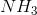), in which the electron-pair geometry is tetrahedral, but the molecular geometry is trigonal pyramidal. As evidenced below:

Ammonia: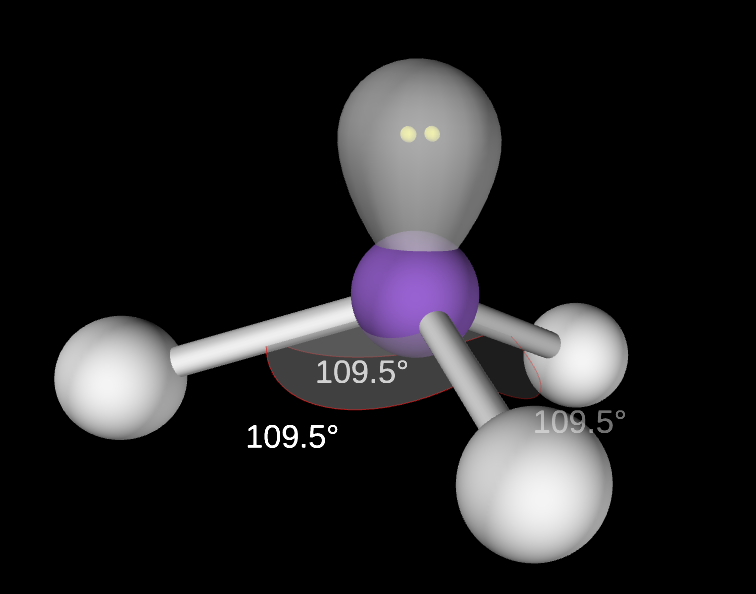Note that the difference in geometry arises in the presence of the lone pair on nitrogen in ammonia. The lone pair only contributes to molecular geometry, but does not contribute to electron-pair geometry. Bonding electrons, however, contribute to both electron and molecular geometries.

Ammonium: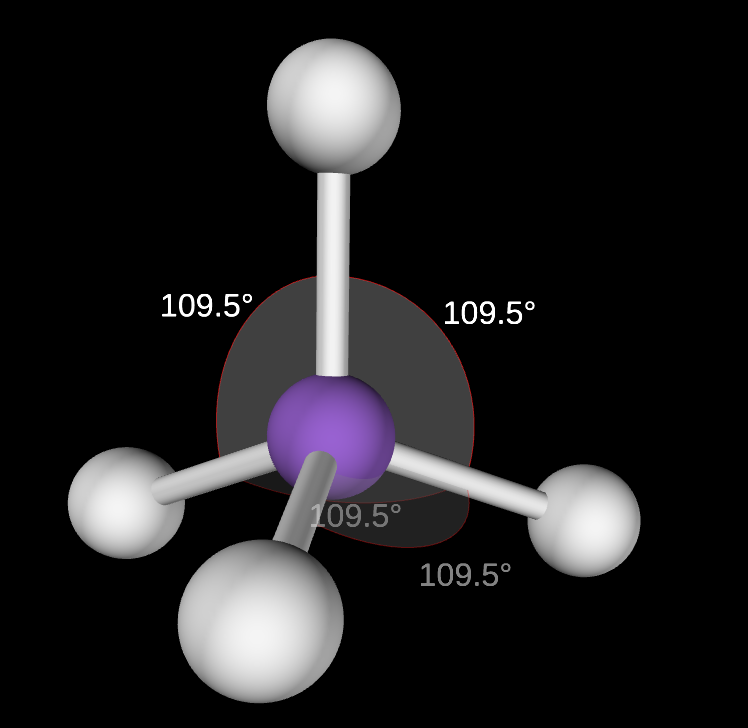In ammonium, the lone pair seen in ammonia is shared in a bond with an additional hydrogen atom.